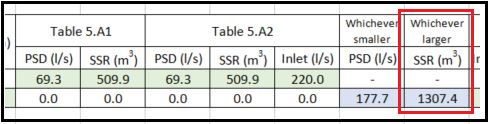603 - 5885 1250 (4 lines) info@mes100.com
Select Page

This is a OSD underground tank design result comparison between manual calculation with MES Software.

In this documentation, a comparison of results between manual calculation and MES Software result will be shown. This comparison will be based on SUStoM requirement which is now part of the submission requirement from DID Sarawak, whereby design engineer will be required to use SUStoM for their project submission.

Comparison between OSD sizing of 30m x 30m x 1.5m RC detention storage tank  by manual calculation with MES Software.

OSD MSMA 2 – Simplified Method

Project file here

Summary Report here

MES SOFTWARE TEXTUAL REPORT VS MANUAL SPREADSHEET

Reference  Calculation  Output
##### 1. Design Criteria

Design Manual = MSMA 2nd Edition

Method = Simplified Method

Type of OSD = Below Ground

Figure 5.A1 2. Proposed Development

Location = Terengganu – Kuala Terengganu

Region = Region 2 – East Coast

Project Area = 2.564 ha

Impervious Area = 1.923 ha

Pervious Area = 0.641 ha

Percentage of Impervious Area = 75%

Terrain = SteepFrom Client’s Manual Calc. Impervious = 75%
Table 5A1 3. Permissible Site Discharge (PSD)

Permissible Site Discharge (PSD)/ha =

For area of 2.564 ha, PSD = 2.564 x 69.30 =

69.30 l/s/ha

177.69 l/s

Table 5A2 Permissible Site Discharge (PSD)/ha =

For area of 2.564 ha, PSD = 2.564 x 69.30 =

69.30 l/s/ha

177.69 l/s

Permissible Site Discharge (PSD) =

(whichever is smaller from Table 5A1 and A2)

177.69 l/sFrom Client’s Manual Calc.Qpost =177.7l/s
Table 5A1 4. Site Storage Requirement (SSR)

Site Storage Requirement (SSR)/ha =

For area of 2.564 ha, SSR = 2.564 x 509.90 =

509.90 m3/ha

1307.38 m3

Table 5A2 Site Storage Requirement (SSR)/ha =

For area of 2.564 ha, SSR = 2.564 x 509.90 =

509.90 m3/ha

1307.38 m3

Site Storage Requirement (SSR) =

(whichever is larger from Table 5A1 and 5A2)

1307.38 m3From Client’s Manual Calc. SSR = 1307.4m3
5. OSD Tank Sizing

The required storage is 1307.38 m3

The dimension for the proposed below-ground storage tank:

V = Length × Width × Depth

= 30.00 × 30.00 × 1.60

= 1440.00 m3From Client’s Manual Calc. = 1350 m3

Table 5A3

6. Inlet & Outlet Sizing

Inlet Pipe

Inlet pipe diameter =

719 mm

Table 5A2 Inlet/ha =

For area of 2.564 ha, Inlet Flow = 2.564 x 220.0/1000 =

220.00 1/s/ha

0.564m3/s

Table 5A4 Inlet Pipe diameter = 786 mm
Required Inlet Pipe diameter =

(whichever smaller from Table 5.A3 and 5.A4)

719 mm
Provide 1 nos. 720 mm diameter pipe 1 Nos. 720 mm
Primary Outlet

Primary outlet discharge should not exceed the lesser PSD flow.

Table  5A3  Outlet pipe diameter = 327mm
Table 5A4 PSD = 177.6851/s =

Outlet pipe diameter =

0.178 m3/s

418 mm

Required Outlet Pipe diameter =

(whichever smaller from Table 5.A3 and 5.A4)

327 mm
Provide 1 nos. 327 mm diameter pipe  1 Nos. 327mm
Ao = No. of Orifice × π × (D/2)2

= 1 × π × (0.327/2)2

= 0.08398m2

Ho = Depth – Diameter/2 – H

Ho = 1.6 – 0.327/2  – 0.900

Ho = 0.537 m

Q = CdAo(2gHo)0.5

= 0.62 × 0.084(2 × 9.81 × 0.5365)0.5

= 0.172m3/s

= 168.93l/s

168.93l/s < 177..685/s

OK

Secondary Outlet
Table 5A3 Required overflow pipe diameter = 719 mm
Ao = π × (D/2)2

= π × (0.719/2)2

= 0.406 m2

Provide 1 nos. 720 mm diameter pipe 1 Nos. 720mm
Ao = No. of Orifice × π × (D/2)2

= 2 × π × (0.720/2)2

= 0.40715m2